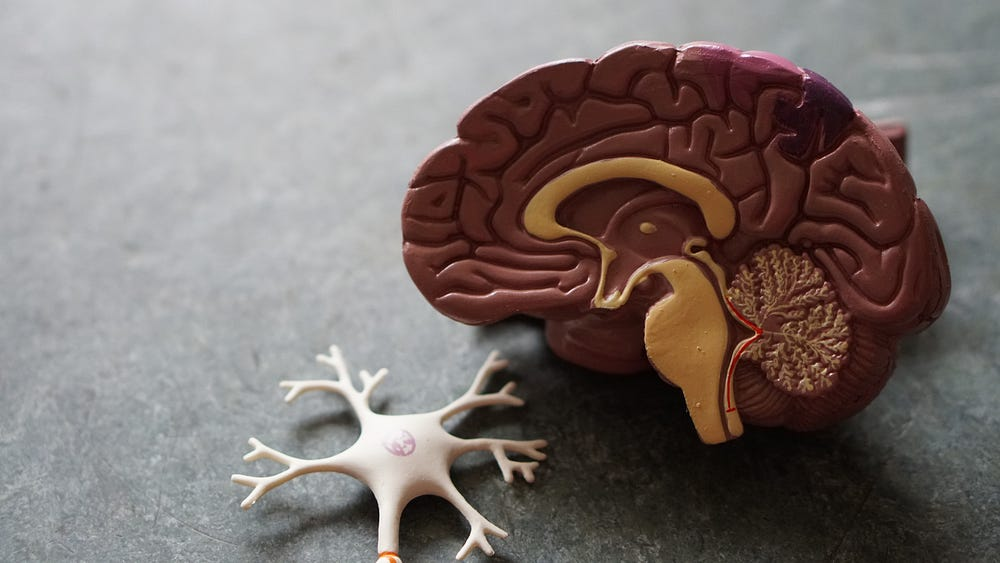# Introduction to Artificial Neural Networks

Original article was published on Deep Learning on Medium# Motivation

Artificial neural networks (ANNs) are really cool systems that help us solve all sorts of problems that would have once seemed unsolvable. ANNs can be used to identify objects in images, understand meaning in texts, forecast profits of businesses, and even perform diagnoses in healthcare.

# Introduction

Depending on who you are and what you know, you might be wondering what an ANN is and what it can do. To introduce ANNs it will be beneficial to first introduce biological neural networks (BNNs), the networks that make our brains and bodies work the way they do.

## Neurons

Below you will find a drawing of a neuron. There are three important parts of the neuron that you will want to focus on:

You can think of the dendrites as input wires, the cell body as a something that performs a computation, and the axon as an output wire. The dendrites bring in information, perform some computation on it, and output the result via the axon.

A biological neural network is what you get when you put together a bunch of neurons.

These networks make it possible to send information all over the body and are the key to how we humans function.

With that being said it is easy to see why one might want to replicate this network.

# ANN Structure

## Basic Structure

Below you can see a basic ANN with two layers:

• The input layer
• The output layer

The input layer below contains three values. Two inputs, `x_1` and `x_2`, and a bias unit with a constant value of 1.

Each input has an associated weight to go along with it. This essentially signifies how much of an effect it will have in the activation function of the next layer. For the input layer we have weights of `w_0`, `w_1`, and `w_2` which correspond to the bias unit, `x_1`, and `x_2`, respectively.

This is where activation functions come in. Activation functions decide what is to be done with the inputs from the previous layer. In the example above we have:

`y = g(w_0*1 + w_1*x_1 + w_2*x_2)`

where `g()` is the activation function for the output layer.

## Deeper Structure

ANNs can become more complex the more layers you put into them. Layers in between the input layer and output layer are called hidden layers.

These deeper ANNs work the same way as the basic structure. Each neuron has an activation function which takes as input a linear combination of the outputs of the previous layer. The product of the output of the activation function of a neuron and its associated weight is then used as input in the next layer.

# Activation Functions

Activation functions are used to decide what a layer does with the outputs of the previous layer. There are many different kinds of activation functions, each useful in different scenarios. Here are a few of the most common activation functions:

• Sigmoid (logistic)
• TanH
• Rectified Linear Unit (ReLU)
• Leaky ReLU
• Softmax

## Sigmoid (logistic)

The sigmoid activation function is useful because of it’s ability to take any value in the set of real numbers and map it to a value between 0 and 1. This is useful when you want to output a probability.

## TanH

The TanH activation function is similar to the sigmoid activation function except it takes a value in the set of real numbers and maps it to a value between -1 and 1. This function is useful in ANNs for its steeper gradient and negative range.

## Rectified Linear Unit (ReLU)

The ReLU activation function is a widely used activation function and has had success in many machine learning applications. One issue with the sigmoid and TanH activation functions above is that as inputs get large, the gradient get small. This is called the vanishing gradient problem. The ReLU activation function solves this issue. The ReLU activation function also allows for fast learning due to its simplicity.

## Leaky ReLU

The leaky ReLU activation function is another widely used activation function. The leaky ReLU activation function was introduced as a way to eliminate the dying ReLU problem, which is the issue with all negative values being mapped to 0 in the ReLU activation function. The Leaky ReLU activation solves this issue while maintaining simplicity, allowing for fast learning.

## Softmax

The softmax activation function is similar to the sigmoid activation function but is used for multi-class classification. The softmax activation function will output as many values as you have classes, each between 0 and 1 and all summing to 1.

# Conclusion

Hopefully from reading this article you were able to understand the fundamentals of neural networks and how they can be used.

If you have any questions or comments feel free to send me a message on LinkedIn.